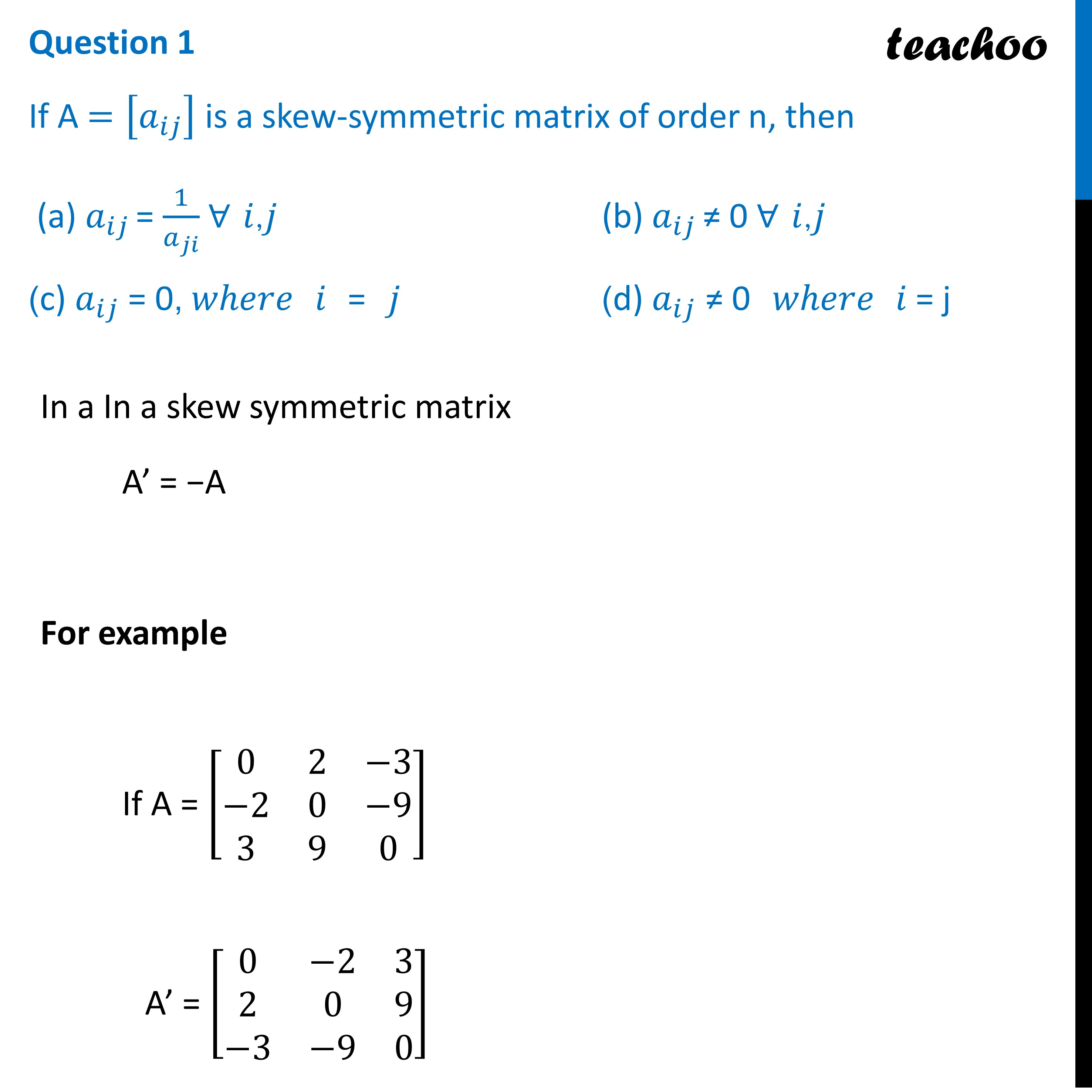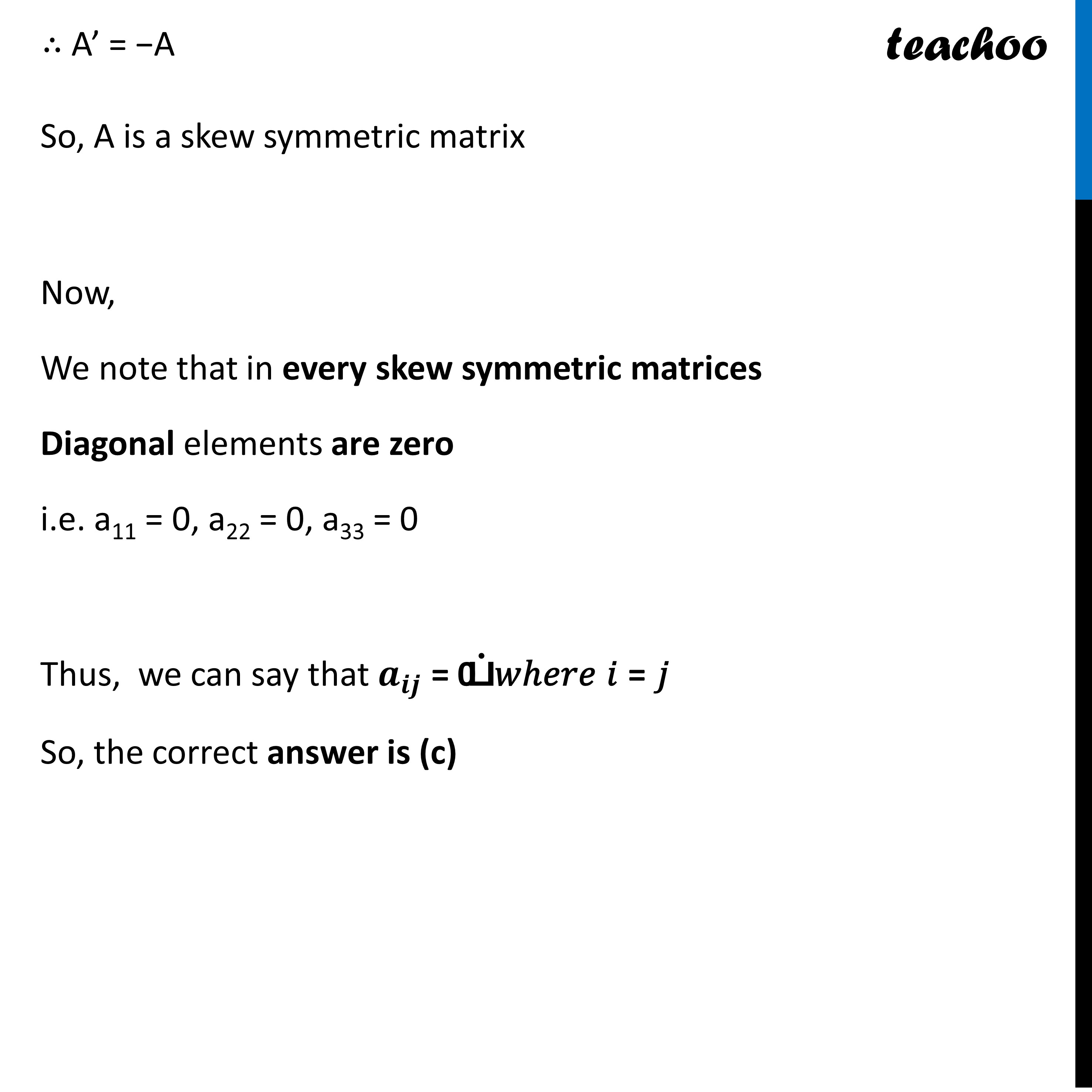CBSE Class 12 Sample Paper for 2023 Boards

Class 12
Solutions of Sample Papers and Past Year Papers - for Class 12 Boards

## (d) a ij ≠ 0 𝑤ℎ𝑒𝑟𝑒 𝑖 = jLearn in your speed, with individual attention - Teachoo Maths 1-on-1 Class

### Transcript

Question 1 If A =[𝑎_𝑖𝑗 ] is a skew-symmetric matrix of order n, then (a) 𝑎_𝑖𝑗 = 1/𝑎_𝑗𝑖 ∀ 𝑖,𝑗 (b) 𝑎_𝑖𝑗 ≠ 0 ∀ 𝑖,𝑗 (c) 𝑎_𝑖𝑗 = 0, 𝑤ℎ𝑒𝑟𝑒 𝑖 = 𝑗 (d) 𝑎_𝑖𝑗 ≠ 0 𝑤ℎ𝑒𝑟𝑒 𝑖 = j In a In a skew symmetric matrix A’ = −A For example If A = [■8(0&2&−3@−2&0&−[email protected]&9&0)] A’ = [■8(0&−2&[email protected]&0&9@−3&−9&0)] ∴ A’ = −A So, A is a skew symmetric matrix Now, We note that in every skew symmetric matrices Diagonal elements are zero i.e. a11 = 0, a22 = 0, a33 = 0 Thus, we can say that 𝒂_𝒊𝒋 = 0, 𝑤ℎ𝑒𝑟𝑒 𝑖 = 𝑗 So, the correct answer is (c)